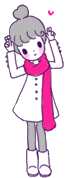ps.是說，如果哪天我們在汗蒸幕的浴池相遇，千萬要裝作不認識，不然我會切腹自殺.

but!!!這怎麼行呢!外頭還有那麼多設施等著我們，(汗蒸幕把毛孔全都打，冰宮可以再把毛孔給縮縮縮回去)((((((((((((((((((((((((((((((((((()))))))))))))))))))))))))))))))))))